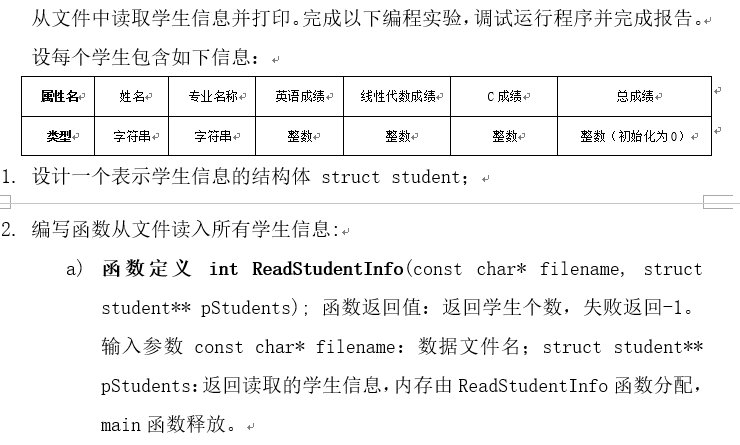b) 建议函数流程：
i. 打开数据文件，失败返回-1；
ii. 从头到尾扫描数据文件（即预读一遍）获得学生人数；
iii. 根据学生人数，利用malloc和sizeof等函数或运算符为struct student** pStudents分配内存；
iv. 利用rewind函数，定位到文件头；
v. 读取数据文件，并把每一条记录通过数组下标法或指针法保存到结构体数组pStudents中；
vi. 关闭文件；
vii. 返回学生人数。

c) 数据文件格式说明：

1. 编写统计函数：
a) 函数定义void Count(struct student pStudents[], int num)。输入参数分别为保存学生的结构体数组和学生人数。
b) 功能为：统计所有学生的总成绩和每个专业（假定一共三个专业：通信，网络，物联网）的平均成绩；输入参数包括学生信息结构体数组，学生人数，返回的每个学生的总成绩直接填充到对应的输入结构体数组对应字段中。统计信息包括三个专业的总人数、平均成绩数组，用全局变量来表示。访问每个学生信息的时候，用指针来指向当前正在处理的学生。
c) 设计一个或者三个带参数的宏，判断输入的字符串是否是指定的专业名称，并在函数Count中使用。

2. 编写main函数实现：
a) 定义变量，其中保存学生信息的数据文件直接在源代码中初始化；
b) 读取学生信息，如果失败，打印失败信息并退出程序；
c) 统计学生信息；
d) 输出统计信息。
e) 释放内存。

``````#include <stdio.h>
#include <stdlib.h>
#include <string.h>

struct student{
char name;
char major;
int Eng_sco;
int cal_sco;
int c_sco;
int sum;
};
int tx_per=0,tx_ave,tx_sum=0;
int wl_per=0,wl_ave,wl_sum=0;
int wlw_per=0,wlw_ave,wlw_sum=0;
int ReadStudentInfo(const char* filename, struct student** pStudents);
void Count(struct student pStudents[], int num);
int main()
{

int count;
int m;
struct student *list;
printf("学生信息： \n");
printf("----------------------------------------------------\n");
printf("序号  姓 名  专业名称 英语成绩 线性代数成绩 c语言成绩 总成绩\n");
for(m=1;m<=count;m++)
{
printf("%4d %s %s %4d %4d %4d %4d\n",m,(list+m-1)->name,(list+m-1)->major,(list+m-1)->Eng_sco,(list+m-1)->cal_sco,(list+m-1)->c_sco,(list+m-1)->sum);
}
Count(list, count);
printf("\n统计信息 ：\n");
printf("----------------------------------------------------\n");
printf("序号  专业名称 学生人数 平均成绩\n");
printf("   1    通信      %d       %d\n",tx_per,tx_ave);
printf("   2    网络      %d       %d\n",wl_per,wl_ave);
printf("   3   物联网     %d       %d\n",wlw_per,wlw_ave);

return 0;
}
int ReadStudentInfo(const char* filename, struct student** pStudents)
{
FILE *fp;
char ch;
int i=1,j;
if(fp==NULL)
{
printf("cann't open the file");
exit(-1);
}
ch=fgetc(fp);
while(ch!=EOF)
{
if(ch=='\n')
{
i++;
}
ch=fgetc(fp);
}
rewind(fp);
*pStudents=(struct student*)malloc(sizeof(** pStudents)*i);
for(j=0;j<i;j++)
{
(*pStudents+j)->sum=((*pStudents+j)->Eng_sco+(*pStudents+j)->cal_sco+(*pStudents+j)->c_sco);
}
fclose(fp);
return i;
}
void Count(struct student pStudents[], int num)
{
int n=1;
for(n=1;n<=num;n++)
{
if(!strcmp((pStudents+n-1)->major,"通信"))
{
tx_per++;
tx_sum=tx_sum+(pStudents+n-1)->sum;
}
if(!strcmp((pStudents+n-1)->major,"网络"))
{
wl_per++;
wl_sum=wl_sum+(pStudents+n-1)->sum;
}
if(!strcmp((pStudents+n-1)->major,"物联网"))
{
wlw_per++;
wlw_sum=wlw_sum+(pStudents+n-1)->sum;
}
tx_ave=tx_sum/tx_per;
wl_ave=wl_sum/wl_per;
wlw_ave=wlw_sum/wlw_per;
}
free(pStudents);
}

``````

13个回答

`````` \#include <stdio.h>
#include <stdlib.h>
#include <string.h>

struct student{
char name;
char major;
int Eng_sco;
int cal_sco;
int c_sco;
int sum;
};
int tx_per=0,tx_ave,tx_sum=0;
int wl_per=0,wl_ave,wl_sum=0;
int wlw_per=0,wlw_ave,wlw_sum=0;
int ReadStudentInfo(const char* filename, struct student** pStudents);
void Count(struct student pStudents[], int num);
int main()
{

int count;
int m;
struct student *list;
printf("学生信息： \n");
printf("----------------------------------------------------\n");
printf("序号  姓名  专业名称  英语成绩   线性代数成绩    c语言成绩   总成绩\n");
for(m=1;m<=count;m++)
{
printf("%4d %6s %6s %4d %4d %4d %4d\n",m,(list+m-1)->name,(list+m-1)->major,(list+m-1)->Eng_sco,(list+m-1)->cal_sco,(list+m-1)->c_sco,(list+m-1)->sum);
}
Count(list, count);
printf("\n统计信息 ：\n");
printf("----------------------------------------------------\n");
printf("序号  专业名称 学生人数 平均成绩\n");
printf("   1    通信      %d       %d\n",tx_per,tx_ave);
printf("   2    网络      %d       %d\n",wl_per,wl_ave);
printf("   3   物联网     %d       %d\n",wlw_per,wlw_ave);

return 0;
}
void CutString(char* buf,char*temp,char sp);
int ReadStudentInfo(const char* filename, struct student** pStudents)
{
FILE *fp;
char ch;
int i=1,j;
fp=fopen(filename,"r");
if(fp==NULL)
{
printf("cann't open the file");
exit(-1);
}
ch=fgetc(fp);
while(ch!=EOF)
{
if(ch=='\n')
{
i++;
}
ch=fgetc(fp);
}
rewind(fp);
*pStudents=(struct student*)malloc(sizeof(** pStudents)*i);

char buf={0};
for(j=0;j<i;j++)
{
memset(*pStudents+j,0,sizeof(struct student));
fgets(buf,512,fp);
int len = strlen(buf);
buf[len]=' ';

CutString(buf,(*pStudents+j)->name,' ');
CutString(buf,(*pStudents+j)->major,' ');
char e_Score,cal_Score,c_Score;

memset(e_Score,0,8);
memset(cal_Score,0,8);
memset(c_Score,0,8);
CutString(buf,e_Score,' ');
(*pStudents+j)->Eng_sco = atoi(e_Score);
CutString(buf,cal_Score,' ');
(*pStudents+j)->cal_sco = atoi(cal_Score);
CutString(buf,c_Score,' ');
(*pStudents+j)->c_sco = atoi(c_Score);
(*pStudents+j)->sum=((*pStudents+j)->Eng_sco+(*pStudents+j)->cal_sco+(*pStudents+j)->c_sco);
}
fclose(fp);
return i;
}

void CutString(char* buf,char*temp,char sp)
{
int len = strlen(buf);
for (int i=0;i<len;i++)
{
if (buf[i]==sp)
{
memcpy(buf,buf+i+1,strlen(buf+i+1));
break;
}
temp[i] = buf[i];
}
}

void Count(struct student pStudents[], int num)
{
int n=1;
for(n=1;n<=num;n++)
{
if(!strcmp((pStudents+n-1)->major,"通信"))
{
tx_per++;
tx_sum=tx_sum+(pStudents+n-1)->sum;
tx_ave=tx_sum/tx_per;
}
if(!strcmp((pStudents+n-1)->major,"网络"))
{
wl_per++;
wl_sum=wl_sum+(pStudents+n-1)->sum;
wl_ave=wl_sum/wl_per;
}
if(!strcmp((pStudents+n-1)->major,"物联网"))
{
wlw_per++;
wlw_sum=wlw_sum+(pStudents+n-1)->sum;
wlw_ave=wlw_sum/wlw_per;
}
}
free(pStudents);
}

``````senlijiu 我这样改，但就是运行不起来。我把fopen中的文件名改为一个不存在的文件名时，可以打印出cann't open the file然后跳出。但改为形参或存在的文件名时，程序就运行不起来
2 年多之前 回复

``````fp=fopen(readme.txt,"r");第一个参数路径需要双引号
``````

fp=fopen(filename,"r");c语言实现txt文件读取

VC编译器下c语言没有办法打开TXT文件。代码如下：#include <stdio.h> #include<stdlib.h> void main() { FILE *fp; if((fp=fopen("C:\Users\Administrator\Desktop\ad.txt","r"))==NULL) { printf("cannot open the file!"); exit(0); } else printf("ok"); fclose(fp); }

C语言fopen打开文件失败

#include <stdio.h> #include <string.h> #include <stdlib.h> //宿舍管理软件 //结构体，主题是学生，包括 struct students{ char name; int id; int dorm_id; int building_id; }student={ {"张三",2019,01,0003}, {"李四",2020,02,0002}, {"王五",2001,03,0001}, {"马六",2003,04,0002}, {"赵七",2018,05,0001} }; void sort_id(); void sort_name(); void sort_drom_id(); typedef struct students stuinfo; //函数体模块 //操作功能页面 void display(){ printf("---------------------------------------\n"); printf("----------请输入要选择的值-------------\n"); printf("----------1:存储学生信息---------------\n"); printf("----------2:排序学生信息---------------\n"); printf("----------3:查找学生信息---------------\n"); printf("----------4:显示学生信息---------------\n"); } //排序 //按照姓名 //按照学号 //按照宿舍号 void display_sort(){ printf("1：按照学号"); printf("2：按照姓名"); printf("3：按照宿舍号"); } void sort(){ char str; int i,j,m; stuinfo temp; //进行排序 printf("1：按照学号"); printf("2：按照姓名"); printf("3：按照宿舍号"); scanf("%d",&m); switch(m){ case 1:{ sort_id(); break; } case 2:{ sort_name(); break; } case 3:{ sort_drom_id(); break; } default :printf("请输入1，2，3中的数字"); } printf("\n 排序已经完成，请按任意键继续\n"); } void sort_id(){ int i,j,m; stuinfo temp; //进行排序 for(i=0;i<5-1;i++) for(j=0;j<5-1-i;j++) if(student[j].id>student[j+1].id) { temp=student[j]; student[j]=student[j+1]; student[j+1]=temp; } printf("按照学号排序完成"); } void sort_name(){ int i,j,m; stuinfo temp; //进行排序 for(i=0;i<5-1;i++) for(j=0;j<5-1-i;j++) if(strcmp(student[j].name,student[j+1].name)>0) { temp=student[j]; student[j]=student[j+1]; student[j+1]=temp; } printf("按照姓名排序完成"); } void sort_drom_id(){ int i,j,m; stuinfo temp; //进行排序 for(i=0;i<5-1;i++) for(j=0;j<5-1-i;j++) if(student[j].dorm_id>student[j+1].dorm_id) { temp=student[j]; student[j]=student[j+1]; student[j+1]=temp; } printf("按照宿舍号排序完成"); } //查找学生信息 int found(){ int low,high,mid,m; low=0; high=5-1; printf("1：按照学号查找"); printf("2: 按照姓名查找"); printf("3: 按照宿舍号查找"); scanf("%d",&m); switch(m){ case 1: { sort_id(); int key; int flag=0; printf("请输入要查找的学号"); scanf("%d",&key); while(low<=high) { mid=(low+high)/2; //计算中间元素序号 if(student[mid].id==key) //中间元素与关键字相等 {return mid; flag=1; } else if(student[mid].id>key) //中间元素大于关键字 high=mid-1; else low=mid+1; } if(flag==0){ return 100; } break; } case 2:{ sort_name(); char key; printf("请输入要查找的姓名");int flag=0; gets(key); while(low<=high) { mid=(low+high)/2; //计算中间元素序号 if(strcmp(student[mid].name,key)==0) //中间元素与关键字相等 {return mid; flag=1; } else if(strcmp(student[mid].name,key)>0) //中间元素大于关键字 high=mid-1; else low=mid+1; } if(flag==0){ return 100; } break; } case 3:{ sort_drom_id(); int key; printf("请输入要查找的宿舍号"); scanf("%d",&key);int flag=0; while(low<=high) { mid=(low+high)/2; //计算中间元素序号 if(student[mid].dorm_id==key) //中间元素与关键字相等 {return mid; flag=1; } else if(student[mid].dorm_id>key) //中间元素大于关键字 high=mid-1; else low=mid+1; } if(flag==0){ return 100; } break; } } } int savetofile() { FILE *fp; if((fp=fopen("stu_info.txt","wb+"))==NULL) //打开文件失败 { printf("无法打开文件！\n"); printf("请按任意键继续\n"); getchar(); return 0; } printf("\n 存文件...\n"); fwrite(student,sizeof(stuinfo)*5,1,fp); //写入文件 fclose(fp); printf("5条记录已经存入文件，请按任意键继续。\n"); //savedflag=0;//更新是否已保存的标记 getchar(); return 1; } int loadfromfile(void) { FILE *fp; int i; if((fp=fopen("stu_info.txt","rb"))==NULL) //打开文件失败 { printf("无法打开文件！\n"); printf("请按任意键继续\n"); getchar(); return 0; } printf("\n 读取文件...\n"); int applicantnum=0; //重新计算员工数 while(!feof(fp)) //读取文件内容 { fread(&student[applicantnum],sizeof(stuinfo),1,fp); applicantnum++; } applicantnum--; fclose(fp); printf("共从文件中读入%d 条记录\n",applicantnum); //p=records; printf("读出的文件内容为：\n"); //showtitle(); //先显示原信息 for(i=0;i<=applicantnum;i++) { printf("姓名：%s 学号：%d 宿舍号：%d 楼号：%d\n",student[i].name,student[i].id,student[i].dorm_id,student[i].building_id); } printf("请按任意键继续！\n"); getchar(); return 1; } //排序 //按照姓名 //按照学号 //按照宿舍号 //打印学生信息 //建立数据文件 //数据文件按照关键字（姓名，学号，宿舍号） //按姓名查询 //按学号查询 //按宿舍号查询 void main(){ int m,n; m=1; int w; while(m){ display(); scanf("%d",&n); switch(n){ case 1:{ printf("存储c语言的文件"); int s=savetofile(); if(s=1){ printf("存储完成"); } break; } case 2:{ sort(); break; } case 3:{ w=found(); if(w==100){ printf("没有查找到"); }else{ printf("查找出来的学生为/n"); printf("姓名：%s 学号：%d 宿舍号：%d 楼号：%d",student[w].name,student[w].id,student[w].dorm_id,student[w].building_id); } break; } case 4:{ loadfromfile(); int i; for(i=0;i<5;i++){ // printf("姓名：%s 学号：%d 宿舍号：%d 楼号：%d",student[i].name,student[i].id,student[i].dorm_id,student[i].building_id); } break; } default:printf("请输入正确的值"); } } } 帮忙可有偿

C++下如何从读取文件内的数据并且排序然后输出到另外一个文件

【C语言】结构体数组作为函数参数的排序问题

springboot+jwt实现token登陆权限认证

MySQL数据库面试题（2020最新版）

2020阿里全球数学大赛：3万名高手、4道题、2天2夜未交卷

HashMap底层实现原理，红黑树，B+树，B树的结构原理 Spring的AOP和IOC是什么？它们常见的使用场景有哪些？Spring事务，事务的属性，传播行为，数据库隔离级别 Spring和SpringMVC，MyBatis以及SpringBoot的注解分别有哪些？SpringMVC的工作原理，SpringBoot框架的优点，MyBatis框架的优点 SpringCould组件有哪些，他们...

Python爬虫，高清美图我全都要（彼岸桌面壁纸）

Java岗开发3年，公司临时抽查算法，离职后这几题我记一辈子

C 语言编程 — 堆栈与内存管理